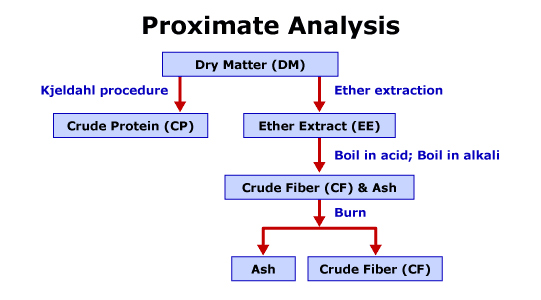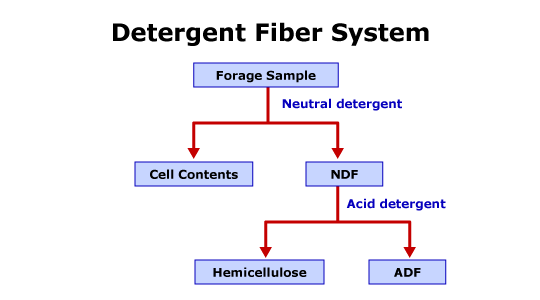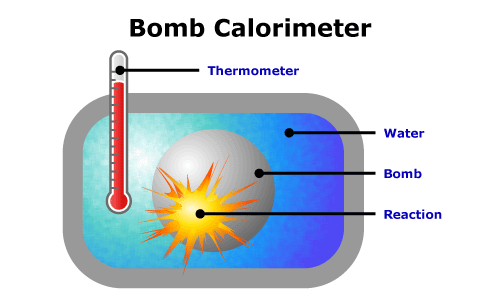## Laboratory-Based Analyses

The primary objectives of laboratory-based analyses are to measure the amount of each individual component in the feedstuff. The components measured are the nutrients. Depending on the specific analysis, the analysis will measure the total amount of the nutrient class or the amount of a specific nutrient within the nutrient class. For example, one analysis will measure the total amount of protein and another analysis will measure the individual amino acids in the feedstuff. The laboratory-based estimations provide an accurate measure of nutrient quantity. However, the laboratory-based estimations do not provide nutrient utilization information.

The initial procedures used to estimate the quantity of an individual component of a feedstuff are the procedures of the proximate analysis system. Each of the procedures provides a quantitative assessment. The system is a series of chemical analyses to estimate the quantity of individual components of a feedstuff. Figure 3.6 is a brief diagram of the proximate analysis system.The initial procedure of proximate analysis is to determine the dry matter (DM) content of the feedstuff. The dry matter procedure removes the free water from the sample. To perform the procedure the original sample is weighed, the weighed sample is placed in a 105 degree Celsius oven for 12-16 hours, and the sample is reweighed. The dry matter and moisture content of the feedstuff are calculated. The second procedure of proximate analysis is to determine the crude protein (CP) content of the feedstuff. The crude protein procedure estimates the quantity of protein and nonprotein nitrogen compounds in the feedstuff. The Kjeldahl method is used to measure the nitrogen content of the sample. The nitrogen content is converted to a crude protein estimate under the assumption all proteins contain approximately 16% nitrogen. The procedure consists of boiling a dried sample in sulfuric acid, the sample is then diluted with water and neutralized with sodium hydroxide, and finally, the sample is distilled and the distilled ammonium is titrated with a known concentrate of sulfuric acid. To determine crude protein content, the nitrogen value from the procedure is multiplied by 6.25. The procedure introduces two primary sources of error. The procedure incorrectly assumes all nitrogen-containing compounds are proteins and all nitrogen sources are 16% nitrogen. The crude fat or ether extract (EE) procedure estimates the quantity of lipids. To perform the procedure, the dried samples are ground and extracted with an organic solvent for 4 hours and the remaining residue is dried and weighed. Ether extract is the calculated as the difference between the original sample and the ether extract residue. The main source of error is the ether extract procedure incorrectly assumes all substances soluble in diethyl ether are lipids. The ash procedure estimates the quantity of inorganic material or minerals, known as ash, in the feedstuff. To perform the procedure, the sample is heated at 500-600 degrees Celsius for 2-4 hours and the remaining residue is weighed. The remaining residue is ash. The crude fiber (CF) procedure estimates hemicellulose, cellulose, lignin, pectin, and other components associated with the fibrous carbohydrates in feedstuffs. The process attempts to simulate the pH-related digestive processes in the stomach and the small intestine of the animal. The process does not account for enzymatic digestion. The sample is prepared by performing the dry matter and subsequently the ether extraction procedure on the sample. To perform the procedure the residue is boiled in a sulfuric acid solution for 30 minutes and rinsed, and then boiled in a sodium hydroxide solution for 30 minutes and rinsed. Finally, the residue is dried, weighed, ashed, and reweighed. The crude fiber content is estimated as the difference between the pre-ash weight and the post-ash weight. The crude fiber procedure has many sources of error and, therefore, is the most unsatisfactory principle of proximate analysis. The primary source of error is that the acidic and basic solutions solubilize some of the true fiber, therefore the procedure underestimates true fiber content of the feedstuff. Another source of error is proteins bound to insoluble fiber fractions are included in the crude fiber content, thus overestimating crude fiber. The inaccuracy of the procedure is the reason laboratories have replaced the crude fiber analysis with the detergent fiber system. The final component of proximate analysis is nitrogen-free extract (NFE). The nitrogen-free extract estimates nonfibrous carbohydrates, such as sugars and starches. The nitrogen free extract determination is the only estimate of proximate analysis determined by a calculation of a difference versus chemical analysis followed by appropriate calculations. The calculation for nitrogen free extract is: % NFE = 100 % – (% EE + % CP + % Ash + % CF). As nitrogen-free extract is calculated by difference, all the errors associated with proximate analysis are additive in the estimate of nitrogen-free extract.

The estimations of carbohydrates in the proximate analysis system, i.e. crude fiber and nonfiber extracts, especially for fibrous feedstuffs, have been replaced with the estimations provided by the detergent fiber system.

Figure 3.7 is a brief diagram of the detergent fiber system.The detergent fiber system more accurately defines the carbohydrate fraction in feedstuffs. The detergent fiber system divides structural carbohydrates into neutral detergent fiber (NDF) and acid detergent fiber (ADF) fractions. The system also estimates nonfibrous carbohydrates (NFC). The procedure uses two detergents to determine the fractions. To perform the NDF procedure, the dried sample is boiled in a neutral solution for 1 hour. The neutral detergent extracts, also referred to as cell wall contents (CWC), are the more digestible fractions of the feedstuffs. The CWC include organic acids, sugars, and other water solubles, lipids, pectins, nonprotein nitrogen sources, and soluble proteins. The insoluble fraction or neutral detergent residue is referred to as neutral detergent fiber. The NDF primarily contains the major cell wall components including hemicellulose, cellulose, and lignin. NDF also contains some minor cell components such as some proteins, bound nitrogen, and minerals. An additional process determines the ADF fraction of forages. The dried NDF residue is boiled in sulfuric acid based solution. The ADF procedure primarily solubilizes hemicellulose and cell wall proteins. Therefore, the ADF residue consists of cellulose, lignin and lignified nitrogen, and other minor cell wall components. The nonfibrous carbohydrate fraction is estimated by a calculated value. NFC is calculated using the following equation: % NFC = 100 % – (% EE + % CP + % Ash + % NDF). As nonfiber carbohydrates are calculated by difference, the errors associated with associated proximate analysis and the detergent fiber system are additive in the estimate.

As discussed in Module II, energy is not a nutrient. Rather, energy is a property possessed by organic compounds such as CHO, proteins, and lipids. Laboratory procedures are able to estimate the chemical energy of a feedstuff. The procedure uses an oxygen bomb calorimeter to estimate the total chemical energy or gross energy (GE) of a feedstuff.

Figure 3.7 is a simple diagram of a bomb calorimeter.To perform the procedure, a weighed sample and oxygen are added to the chamber, the sample is completely oxidized, and the change in the temperature of the water surrounding the chamber is measured. The gross energy is the total amount of heat produced. The procedure determines the gross energy of the feedstuff; however the gross energy is an inconsistent measure of the energy available to the animal. Therefore, gross energy alone is of little value. However, as discussed later in Module III, gross energy is useful in combination with additional procedures.

In addition to the laboratory procedures described above, there are specialized procedures to briefly discuss. Only the most extensively used specialized procedures will be discussed. The procedures may be used in specific situations such as animal nutrition research, ration formulation for specific animals, and in-the-field problem-solving. The first procedure is high performance liquid chromatography (HPLC). HPLC identifies individual amino acids in a feedstuff. The second procedure is atomic absorption spectrophotometry. Atomic absorption spectrophotometry identifies individual minerals present in a feedstuff. One application of atomic absorption spectrophotometry is to identify mineral contamination of a forage. The third procedure is near-infrared reflectance spectroscopy (NIRS). NIRS is used to perform standard feedstuff analyses. The major disadvantages of NIRS are the high cost and increased potential for inaccurate analyses for mixed feeds and forages. The fourth procedure is gas-liquid chromatography (GLC). Gas-liquid chromatography is used to analyze gaseous compounds or any compound that can be vaporized. One application of GLC is to identify the individual volatile fatty acids.